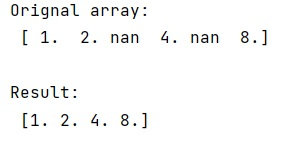# How to remove NaN values from a given NumPy array?

In this tutorial, we will learn how to remove NaN values from a given NumPy array? By Pranit Sharma Last updated : May 23, 2023

Suppose we need to create a NumPy array of length n, and each element of this array would be e a single value (say 5).

## Removing NaN values from a given NumPy array

To remove NaN values from a given NumPy array, you can use a method provided by NumPy called the full() method. This method is better than the empty() followed by the fill() method.

This is arguably the way of creating an array filled with certain values because it explicitly describes what is being achieved.

Let us understand with the help of an example,

## Python program to remove NaN values from a given NumPy array

```# Import numpy
import numpy as np

# Creating a numpy array
arr = np.array([1, 2, np.NaN, 4, np.NaN, 8])

# Display original array
print("Orignal array:\n",arr,"\n")

# Extracting non null values
res = arr[~np.isnan(arr)]

# Display Result
print("Result:\n",res,"\n")
```

### Output